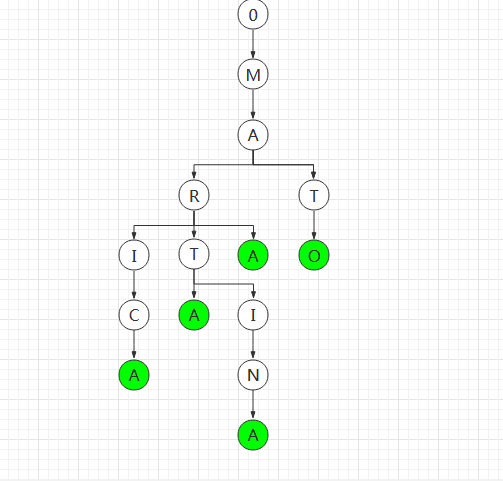IC_QQQ

M32_模拟赛总结

2019-10-24 16:13:48

# M32_模拟赛总结

## T1:AERODROM

### 时空限制

Time Limit: 1000MS

Memory Limit: 32768KB

### 题目：

N个登机口，办理登机业务，第i个窗口的单位办理时间为Ti，M个人办理登机业务，他们可以选择最佳的方案，不考滤换人和换窗口的时间，所有窗口是同时计时的，即同时开始办理业务，请输出所有人都登机的最少时间。如样例1： 2个窗口，6个人，第一个窗口的单位时间是7,第二个是10, 一二个人分别在两个窗口办理，7秒时第三个人可在第一个窗口开始办理，10秒时，第四人开始在窗口二办理，时间14时，第五人一窗口。在时间20，窗口2可以使用，如果第六人在此办理，总时间将是30秒，如果等1秒在一窗口办理，则总时间是28秒。

7 10

3 8 3 6 9 2 4

8

### 代码：

#include <bits/stdc++.h>
#define ll long long
using namespace std;
const int N=1e5+5;
int n,m;ll mp[N],mis=1e9;

ll in(){
ll x=0;char ch=getchar();
while(ch>'9'||ch<'0') ch=getchar();
while(ch>='0'&&ch<='9') x=x*10+ch-'0',ch=getchar();
return x;
}

bool check(ll limit){
int t=0;
for(int i=1;i<=n;i++){
t+=limit/mp[i];
if(t>=m) return true;
}
return false;
}

int main(){
freopen("aerodrom.in","r",stdin);
freopen("aerodrom.out","w",stdout);
n=in(),m=in();
for(int i=1;i<=n;i++)
mp[i]=in(),mis=min(mis,mp[i]);
ll l=0,r=mis*m;
while(l<r){
ll mid=(l+r)>>1;
if(check(mid)) r=mid;
else l=mid+1;
}
printf("%lld\n", l);
return 0;
}


## T2:HERKABE

### 时空限制：

Time Limit: 1000MS

Memory Limit: 32768KB

5

MARICA

MARTA

MATO

MARA

MARTINA

24

4

A

AA

AAA

AAAA

8

### 解析：### 代码：

#include <bits/stdc++.h>
#define ll long long
using namespace std;
const int N=3005,M=1e5+5;
const ll mod=1e9+7;
int n,m;
ll ans=1,A;
string mp[N];

void solve(int pos,int l,int r){//pos:深度
if(l==r) return ;//只有这一个单词，返回
int sum=0,last=l;//sum:字数个数
for(int i=l;i<=r;i++){
if(mp[i][pos]!=mp[last][pos]){//遇到了新的子节点，分治
solve(pos+1,last,i-1);
last=i,sum++;
}
if(i==r) sum++,solve(pos+1,last,i);
}
ans*=A[sum],ans%=mod;
return ;
}

int main(){
freopen("herkabe.in","r",stdin);
freopen("herkabe.out","w",stdout);
ios::sync_with_stdio(false);
cin>>n;
for(int i=1;i<=n;i++)
cin>>mp[i],mp[i]+='*';//处理空字符的情况
sort(mp+1,mp+1+n);
A=1;
for(int i=1;i<=30;i++)
A[i]=(A[i-1]*i)%mod;//阶乘预处理
solve(0,1,n);
cout<<ans%mod;
return 0;
}

## T3:PROCESOR

### 时空限制：

Time Limit: 1000MS

Memory Limit: 32768KB

### 题目：### input

3 3 2 1 2 1 2 1 3 2 2 2 3 3

0 1 2

4 6

2 4 2

3

2 4 1

6

1 3 1

2 3 1

2

1 2 2

2 2 3

7

5 0 14 3

5 6

2 4 2

10

2 5 3

2

2 2 3

1

2 1 4

3

1 3 1

2 3 4

2147483663

15 6 7 12 5

### 解析：

1. w=1，说明 $A_i!=B_i$ ， 如果 $A_i=0$ ， 那么 $B_i=1$ ；如果 $A_i=1$ ， 那么 $B_i=0$ 。

2. w=0，说明 $A_i=B_i$ ， 如果 $A_i=0$ ， 那么 $B_i=0$ ；如果 $A_i=1$ ， 那么 $B_i=1$ 。

1. w=1，说明 $A_i!=B_i$ ， $merge(A_{i0},B_{i1})$ ， $merge(A_{i1},B_{i0})$ 。

2. w=0，说明 $A_i=B_i$ ， $merge(A_{i0},B_{i0})$ ， $merge(A_{i1},B_{i1})$ 。

1. $f_0$ 在 0域 且 $y_{0i}$ 有值，说明 $x_i=y_{0i}$ 。
2. $f_0$ 在 1域 且 $y_{0i}$ 有值，说明 $x_i!=y_{0i}$ 。
3. $f_1$ 在 0域 且 $y_{1i}$ 有值，说明 $x_i!=y_{1i}$ 。
4. $f_1$ 在 1域 且 $y_{1i}$ 有值，说明 $x_i=y_{1i}$ 。

### 代码：

#include <bits/stdc++.h>
using namespace std;
const int N=1e5+5;
int n,m;
int fa[N*32*2];
//0~31是0域，32~63是1域
char mp[N*32*2],move[N];

int find(int x){
return x==fa[x]?x:fa[x]=find(fa[x]);
}

void merge(int u,int v){
fa[find(u)]=find(v);
return ;
}

int main(){
freopen("procesor.in","r",stdin);
freopen("procesor.out","w",stdout);
// ios::sync_with_stdio(false);
int op,a,b;unsigned int c;
cin>>n>>m;
for(int i=1;i<=n;i++){
for(int j=0;j<32;j++){
mp[(i-1)*32*2+j]=-1;
fa[(i-1)*32*2+j]=(i-1)*32*2+j;
fa[(i-1)*32*2+j+32]=(i-1)*32*2+j+32;
}
}
for(int i=1;i<=m;i++){
cin>>op>>a>>b;
if(op==1){
move[a]+=b,move[a]%=32;
}
else{
cin>>c;
for(int j=0;j<32;j++){
int u=(j+move[a])%32;
int v=(j+move[b])%32;
if(c&1){
merge((a-1)*32*2+u,(b-1)*32*2+v+32);
merge((a-1)*32*2+u+32,(b-1)*32*2+v);
}
else{
merge((a-1)*32*2+u,(b-1)*32*2+v);
merge((a-1)*32*2+u+32,(b-1)*32*2+v+32);
}
c>>=1;
}
}
}
for(int i=1;i<=n;i++){
for(int j=31;j>=0;j--){
int f0=find(fa[(i-1)*32*2+j]);
int f1=find(fa[(i-1)*32*2+j+32]);
int p0=f0%64,p1=f1%64;
if(f0==f1){ cout<<"-1"; return 0; }
if(p0<32&&mp[f0]>=0){
mp[(i-1)*32*2+j]=mp[f0];
continue;
}
if(p0>=32&&mp[f0-32]>=0){
mp[(i-1)*32*2+j]=(mp[f0-32]^1);
continue;
}
if(p1>=32&&mp[f1-32]>=0){
mp[(i-1)*32*2+j]=mp[f1-32];
continue;
}
if(p1<32&&mp[f1]>=0){
mp[(i-1)*32*2+j]=(mp[f1]^1);
continue;
}
mp[(i-1)*32*2+j]=0;
if(p0<32) mp[f0]=0;
if(p0>=32) mp[f0-32]=1;
if(p1>=32) mp[f1-32]=0;
if(p1<32) mp[f1]=1;
}
}
for(int i=1;i<=n;i++){
unsigned int ans=0;
for(int j=0;j<32;j++)
ans|=(mp[(i-1)*32*2+j]<<j);
cout<<ans<<" ";
}
return 0;
}

• star
首页